## Machine Learning

A collection of articles on Machine Learning that teach you the concepts and how they’re implemented in practice.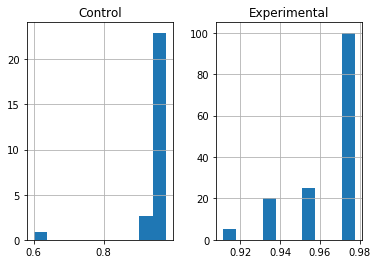## Overview

This will be the fourth article in a four-part series covering the following:

1. Dataset analysis - We will present and discuss a dataset selected for our machine learning experiment. This will include some analysis and visualisations to give us a better understanding of what we're dealing with.

2. Experimental design - Before we conduct our experiment, we need to have a clear idea of what we're doing. It's important to know what we're looking for, how we're going to use our dataset, what algorithms we will be employing, and how we will determine whether the performance of our approach is successful.

3. Implementation - We will use the Keras API on top of TensorFlow to implement our experiment. All code will be in Python, and at the time of publishing everything is guaranteed to work within a Kaggle Notebook.

4. Results - Supported by figures and statistics, we will have a look at how our solution performed and discuss anything interesting about the results.

## Results

In the last article we prepared our dataset such that it was ready to be fed into our neural network training and testing process. We then built and trained our neural network models using Python and Keras, followed by some simple automation to generate thirty samples per arm of our experiment. Now, we'll have a look at how our solutions performed and discuss anything interesting about the results. This will include some visualisation, and we may even return to our experiment code to produce some new results.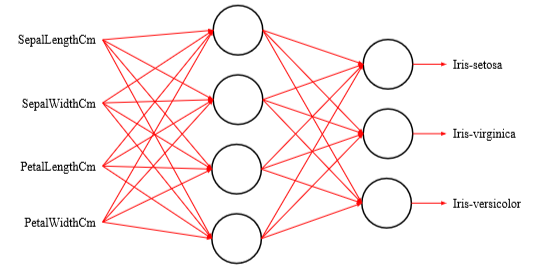Let's remind ourselves of our testable hypothesis:

Hypothesis : A neural network classifier's performance on the Iris Flower dataset is affected by the number of hidden layer neurons.

When we test our hypothesis, there are two possible outcomes:

• ${H}_{0}$ the null hypothesis : insufficient evidence to support hypothesis.

• ${H}_{1}$ the alternate hypothesis : evidence suggests the hypothesis is likely true.

Strictly speaking, our experiments will not allow us to decide on an outcome. Our experimental arm uses the same structure to the control arm except for one variable, and that is the number of neurons on the hidden layer changing from four to five. Therefore, we are only testing to see if this change affects the performance of the neural network classifier.

Similar to the last three parts of this series, we will be using a Kaggle Kernel notebook as our coding environment. If you have saved your files to a file using a Kaggle Notebook, then you will need to load the data file into your draft environment as a data source. It’s not immediately obvious where the files have been stored, but you can locate them by repeating the following steps: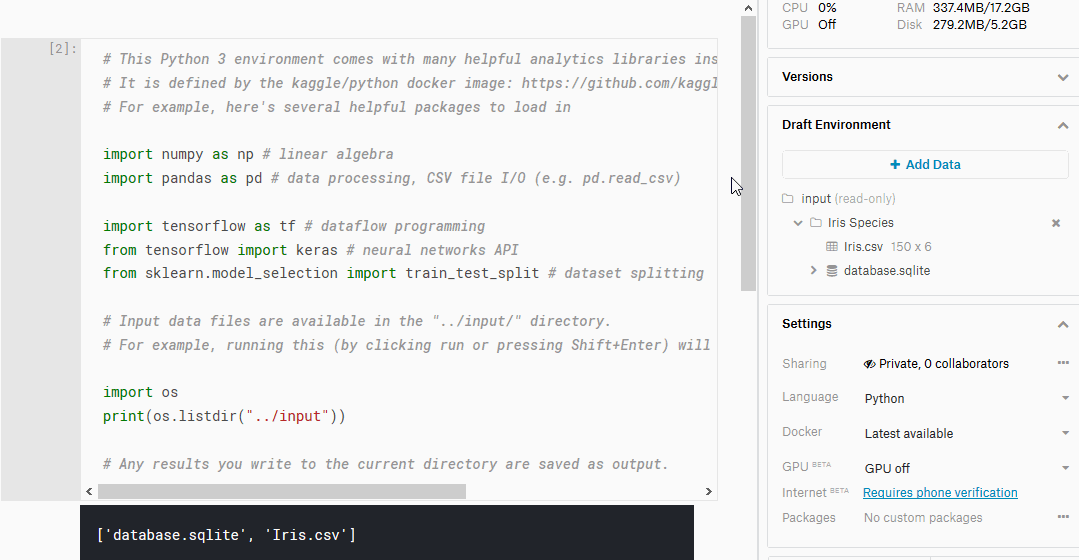Once you have the data in your environment, use the following code to load the data into variables. You will need to adjust the parameters for read_csv() to match your filenames.

Note

Below you will see iris-flower-dataset-classifier-comparison used in the pathnames, be sure to use the correct pathnames for your own experiment.

results_control_accuracy = pd.read_csv("/kaggle/input/iris-flower-dataset-classifier-comparison/results_control_accuracy.csv")



If you don't have access to the results generated from the previous article, then you are welcome to use my results with the following code:

results_control_accuracy = pd.DataFrame([0.9333333359824286, 0.9777777791023254, 0.9777777791023254, 0.9777777791023254, 0.9777777791023254, 0.9777777791023254, 0.9777777791023254, 0.9777777791023254, 0.9555555568801032, 0.9777777791023254, 0.9777777791023254, 0.9777777791023254, 0.9777777791023254, 0.6000000052981906, 0.9777777791023254, 0.9777777791023254, 0.9777777791023254, 0.9111111124356588, 0.9777777791023254, 0.9777777791023254, 0.9777777791023254, 0.9777777791023254, 0.9777777791023254, 0.9777777791023254, 0.9555555568801032, 0.9777777791023254, 0.9777777791023254, 0.9777777791023254, 0.9777777791023254, 0.9111111124356588])

results_experimental_accuracy = pd.DataFrame([0.9111111124356588, 0.9555555568801032, 0.9555555568801032, 0.9777777791023254, 0.9777777791023254, 0.9777777791023254, 0.9555555568801032, 0.933333334657881, 0.9777777791023254, 0.9777777791023254, 0.9777777791023254, 0.9555555568801032, 0.9777777791023254, 0.933333334657881, 0.9777777791023254, 0.9777777791023254, 0.9777777791023254, 0.9777777791023254, 0.9777777791023254, 0.9777777791023254, 0.9777777791023254, 0.9777777791023254, 0.9333333359824286, 0.9777777791023254, 0.9777777791023254, 0.9333333359824286, 0.9777777791023254, 0.9555555568801032, 0.9777777791023254, 0.9777777791023254])


### Basic stats

With our results loaded, we can get some quick stats to start comparing the performance differences between the two arms of our experiment.

We can start by comparing the mean performance of the control arm against the experimental arm. Using pandas and numpy , we can write the following code:

mean_control_accuracy = results_control_accuracy.mean()
print("Mean Control Accuracy: {}".format(mean_control_accuracy))

mean_experimental_accuracy = results_experimental_accuracy.mean()
print("Mean Experimental Accuracy: {}".format(mean_experimental_accuracy))


The output of which will be the following if you've used the data provided above: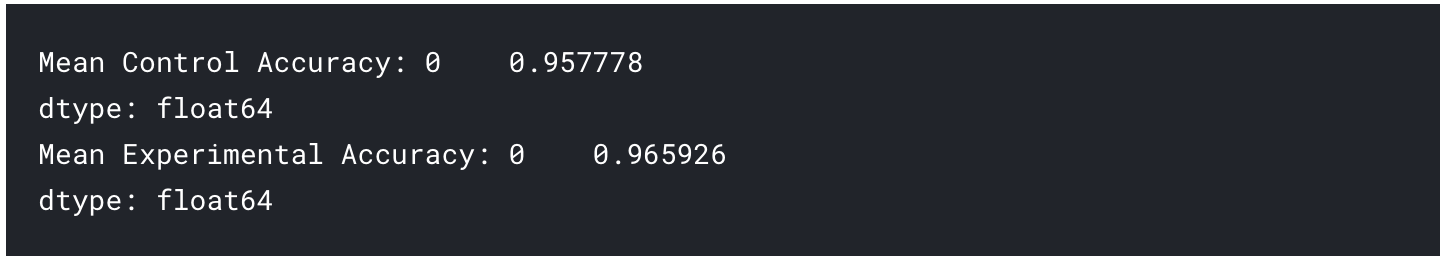At this point, it may be tempting to claim that the results generated by the experimental arm of our experiment has outperformed that of the control arm. Whilst it's true that the mean accuracy of the 30 samples from the experimental arm is higher than the control arm, we are not yet certain of the significance of these results. At this point, this difference in performance could have occurred simply by chance, and if we generate another set of 30 samples the results could be the other way around.

Before moving on, it may also be useful to report the standard deviation of the results in each arm of the experiment:

std_control_accuracy = results_control_accuracy.std()
print("Standard Deviation of Control Accuracy Results: {}".format(std_control_accuracy))

std_experimental_accuracy = results_experimental_accuracy.std()
print("Standard Deviation of Experimental Accuracy Results: {}".format(std_experimental_accuracy))


The output of which will be the following if you've used the data provided above: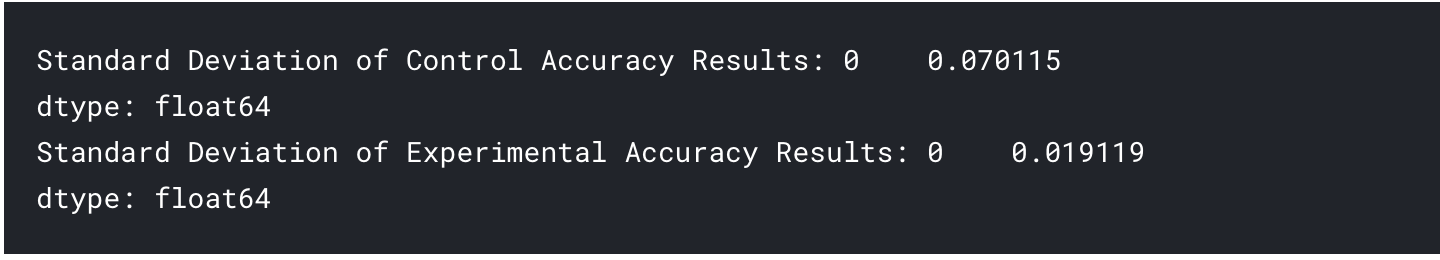### Visualising the results

Moving onto visualisations, one common plot used to compare this type of data is the box plot, which we can produce using pandas.DataFrame.boxplot(). Before we do this, we need to move the results from both arms of our experiment into a single DataFrame and name the columns.

results_accuracy= pd.concat([results_control_accuracy, results_experimental_accuracy], axis=1)
results_accuracy.columns = ['Control', 'Experimental']


If we print out this new variable, we can see all our results are now in a single DataFrame with appropriate column headings: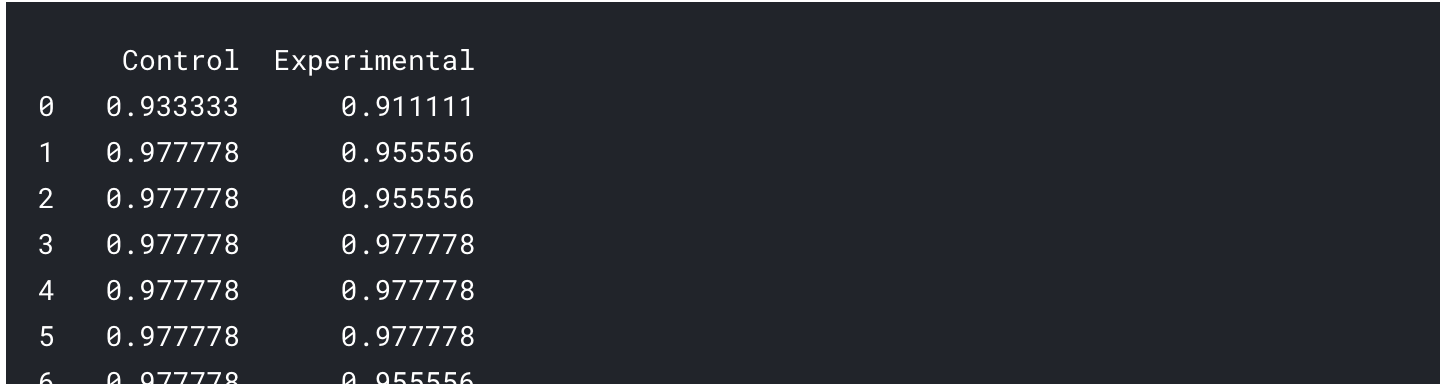We can produce a box plot using a single line of code:

results_accuracy.boxplot()


The output of which will be the following if you've used the data provided above: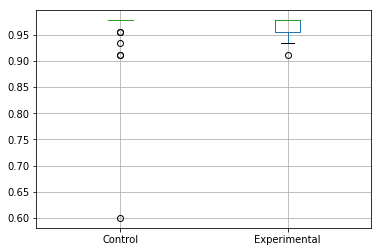However, the scale of the plot has made it difficult to compare the two sets of data. We can see the problem with our own eyes, and it's down to one of the samples from the Control arm of the experiment. One of the samples appears to be around $0.60$ which is a clear outlier.

With pandas we can try two approaches to remove this outlier from view and get a better look. One is not to plot the outliers using the showfliers parameter for the box plot method:

results_accuracy.boxplot(showfliers=False)


Which will output: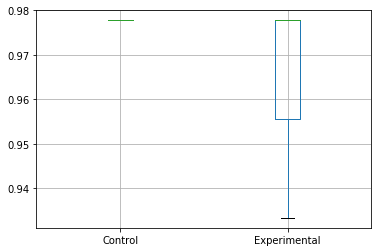Or to instead specify the y-axis limits for the box plot:

ax = results_accuracy.boxplot()
ax.set_ylim([0.9,1])


Which will output: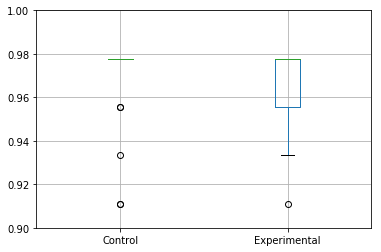### Distribution of the data

It may also be useful to find out if our results are normally distributed, as this will also help us decide what parametric or non-parametric tests to use. You may be able to make this decision using a histogram:

results_accuracy.hist(density=True)


Which will output the following:But normally you will want to use a function that will test the data for you. One approach is to use scipy.stats.normaltest():

This function tests the null hypothesis that a sample comes from a normal distribution.

https://docs.scipy.org/doc/scipy/reference/generated/scipy.stats.normaltest.html

This function will return two variables, one called the statistic and most importantly for us, the p-value , which is the probability of the hypothesis test. A p-value , always between 0 and 1, indicates the strength of evidence against the null hypothesis. A smaller p-value indicates greater evidence against null hypothesis, whilst a larger p-value indicates weaker evidence against the null hypothesis.

For this test, the null hypothesis is that the samples do not come from a normal distribution. Before using the test, we need to decide on a value for alpha , our significance level. This is essentially the “risk” of concluding a difference exists when it doesn’t, e.g., an alpha of $0.05$ indicates a 5% risk. We can consider alpha to be some kind of threshold. This will be covered in more detail in another article, but for now we will set $0.05$ as our alpha. This means if our p-value is less than $0.05$, the null hypothesis has been rejected and the samples are likely not from a normal distribution. Otherwise, the null hypothesis cannot be rejected, and the samples are likely from a normal distribution.

Let's write some code to determine this for us:

from scipy import stats

alpha = 0.05;

s, p = stats.normaltest(results_control_accuracy)
if p < alpha:
print('Control data is not normal')
else:
print('Control data is normal')

s, p = stats.normaltest(results_experimental_accuracy)
if p < alpha:
print('Experimental data is not normal')
else:
print('Experimental data is normal')


The output of which will be the following if you've used the data provided above: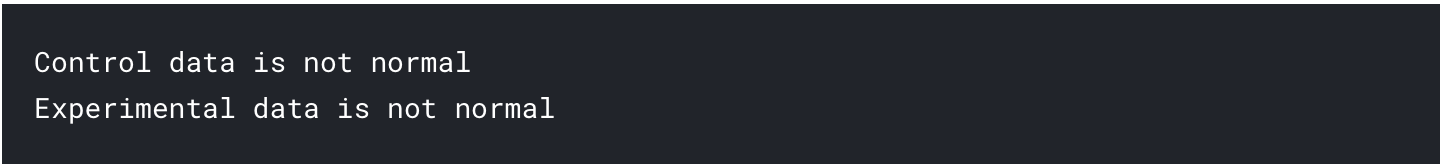#### Significance testing

Finally, let's test the significance of our pairwise comparison. The significance test you select depends on the nature of your data-set and other criteria, e.g. some select non-parametric tests if their data-sets are not normally distributed. We will use the Wilcoxon signed-rank test through the following function: scipy.stats.wilcoxon():

The Wilcoxon signed-rank test tests the null hypothesis that two related paired samples come from the same distribution. In particular, it tests whether the distribution of the differences x - y is symmetric about zero. It is a non-parametric version of the paired T-test.

https://docs.scipy.org/doc/scipy/reference/generated/scipy.stats.wilcoxon.html

This will give us some idea as to whether the results from the control arm are significantly different from those of the experimental arm. This will again return a p-value , and we will compare it with an alpha of $0.05$.

s, p = stats.wilcoxon(results_control_accuracy, results_experimental_accuracy)

if p < 0.05:
print('null hypothesis rejected, significant difference between the data-sets')
else:
print('null hypothesis accepted, no significant difference between the data-sets')


The output of which will be the following if you've used the data provided above: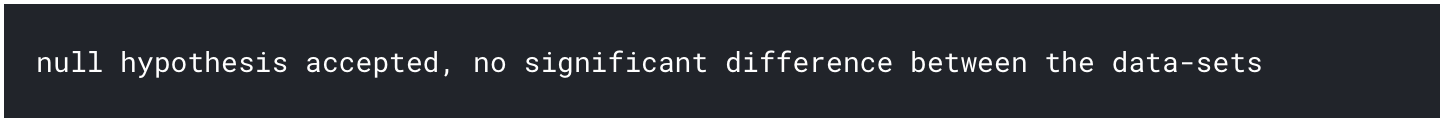This means that although the mean accuracy of our experimental arm samples outperforms the mean performance of our control arm, this is likely to be purely by chance. We cannot say that one is better than the other.

## Conclusion

In the article we had a look at how our solutions performed and using some simple statistics and visualisations. We also tested whether our results came from a normal distribution, and whether results from both arms of our experiment were significantly different from each other. Through significance testing we determined that we were not able to claim that one arm of the experiment outperformed the other, despite the mean performances being different. Regardless, a result is a result, and we can extend our experiment to include a range of neurons per hidden layer instead of four compared five.

Thank you for following this four-part series on Machine Learning with Kaggle Notebooks. If you notice any mistakes or wish to make any contributions, please let me know either using the comments or by e-mail.